Download the eSaral app and start learning from Kota's top IITians and doctors.

# Ray Optics - JEE Main Previous Year Questions with Solutions

JEE Main Previous Year Question of Physics with Solutions are available here. Practicing JEE Main Previous Year Papers Questions of Physics will help all the JEE aspirants in realizing the question pattern as well as help in analyzing their weak & strong areas. Get detailed Class 11th &12th Physics Notes to prepare for Boards as well as competitive exams like IIT JEE, NEET etc. eSaral helps the students in clearing and understanding each topic in a better way. eSaral is providing complete chapter-wise notes of Class 11th and 12th both for all subjects. Besides this, eSaral also offers NCERT Solutions, Previous year questions for JEE Main and Advance, Practice questions, Test Series for JEE Main, JEE Advanced and NEET, Important questions of Physics, Chemistry, Math, and Biology and many more. Download eSaral app for free study material and video tutorials.   Simulator Previous Years AIEEE/JEE Mains Questions
Q. A transparent solid cyclindrical rod has a refractive index of . It is surrounded by air. A light ray is incident at the mid-point of one end of the rod as shown in the figure. The incident angle $\theta$ for which the light ray grazes along the wall of the rod is :-( 1) $\sin ^{-1}\left(\frac{2}{\sqrt{3}}\right)$ (2) $\sin ^{-1}\left(\frac{1}{\sqrt{3}}\right)$ (3) $\sin ^{-1}\left(\frac{1}{2}\right)$ ( 4) $\sin ^{-1}\left(\frac{\sqrt{3}}{2}\right)$ [AIEEE - 2009]
Ans. (2)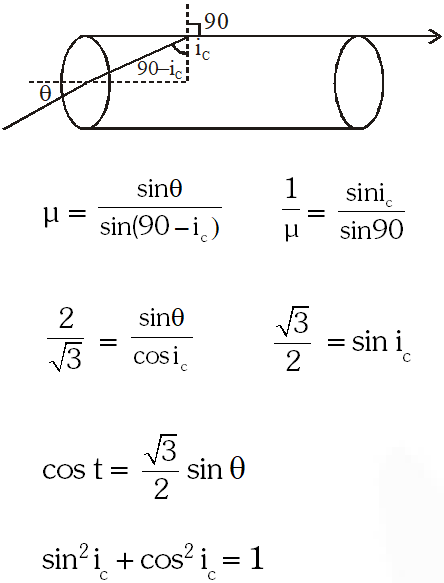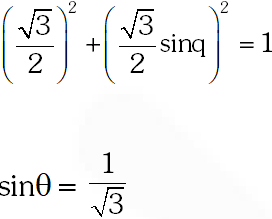Q. A car is fitted with a convex side-view mirror of focal length 20 cm. A second car 2.8 m behind the first car is overtaking the first car at a relative speed of 15 m/s. The speed of the image of the second car as seen in the mirror of the first one is:- (1) 10 m/s (2) 15 m/s (3) $\frac{1}{10} \mathrm{m} / \mathrm{s}$ (4) $\frac{1}{15} \mathrm{m} / \mathrm{s}$ [AIEEE- 2011]
Ans. (4) $V_{i z f r}=-\left[\frac{f}{f-u}\right]^{2} V_{\text {olrq }}=-\left[\frac{20}{20+280}\right]^{2} \times 15$
Q. When monochromatic red light is used instead of blue light in a convex lens, its focal length will :- (1) Does not depend on colour of light (2) Increase (3) Decrease (4) Remain same [AIEEE-2011]
Ans. (2)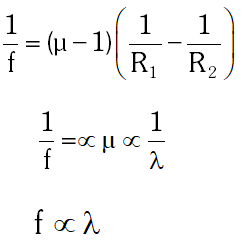Q. A beaker contains water up to a height $\mathrm{h}_{1}$ and kerosene of height $\mathrm{h}_{2}$ above watger so that the total height of (water + kerosene) is $\left(\mathrm{h}_{1}+\mathrm{h}_{2}\right)$. Refractive index of water is $\mu_{1}$ and that of kerosene is $\mu_{2}$. The apparent shift in the position of the bottom of the beaker when viewed from above is :- $(1)\left(1-\frac{1}{\mu_{1}}\right) \mathrm{h}_{2}+\left(1-\frac{1}{\mu_{2}}\right) \mathrm{h}_{1}$ (2) $\left(1+\frac{1}{\mu_{1}}\right) \mathrm{h}_{1}-\left(1+\frac{1}{\mu_{2}}\right) \mathrm{h}_{2}$ (3) $\left(1-\frac{1}{\mu_{1}}\right) \mathrm{h}_{1}+\left(1-\frac{1}{\mu_{2}}\right) \mathrm{h}_{2}$ $(4)\left(1+\frac{1}{\mu_{1}}\right) \mathrm{h}_{2}-\left(1+\frac{1}{\mu_{2}}\right) \mathrm{h}_{1}$ [AIEEE- 2011]
Ans. (3)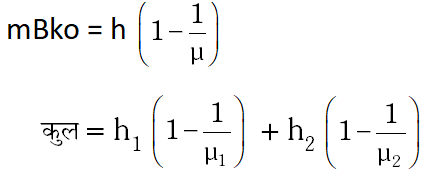Q. An object 2.4 m in front of a lens forms a sharp image on a film 12 cm behind the lens. A glass plate 1 cm thick, of refractive index 1.50 is interposed between lens and film with its plane faces parallel to film. At what distance (from lens) should object be shifted to be in sharp focus on film ? (1) 5.6 m (2) 7.2 m (3) 2.4 m (4) 3.2 m [AIEEE- 2012]
Ans. (1)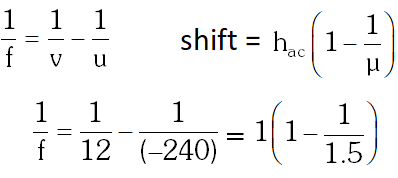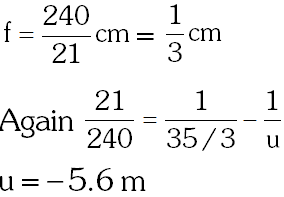Q. Diameter of a plano-convex lens is 6cm and thickness at the centre is 3 mm. If speed of light in material of lens is $2 \times 10^{8} \mathrm{m} / \mathrm{s}$, the focal length of the lens is : (1) 15 cm (2) 20 cm (3) 30 cm (4) 10 cm [JEE-Mains- 2013]
Ans. (3) $\frac{1}{\mathrm{f}}=(\mu-1)\left(\frac{1}{\mathrm{R}_{1}}-\frac{1}{\mathrm{R}_{2}}\right)=\left(\frac{3 \times 10^{8}}{2 \times 10^{8}}-1\right)\left(\frac{1}{15}-\frac{1}{\infty}\right)$ f = 30 cm
Q. The graph between angle of deviation $(\delta)$ and angle of incidence (i) for a triangular prism is represented by :-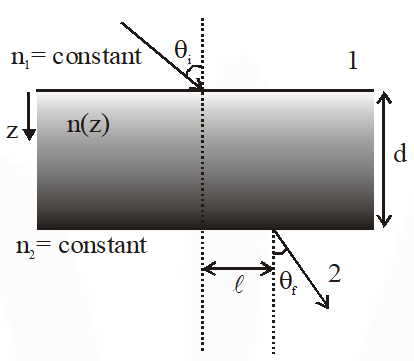[JEE-Mains- 2013]
Ans. (3) Refer NCERT
Q. A thin convex lens made from crown glass $\left(\mu=\frac{3}{2}\right)$ has focal length ƒ. When it is measured in two different liquids having refractive indices $\frac{4}{3}$ and $\frac{5}{3}$ and , it has the focal length $f_{1}$ and $f_{2}$ respectively. The correct relation between the focal lengths is : (1) $f_{2}>f$ and $f_{1}$ becomes negative (2) $f_{1}$ and $f_{2}$ both become negative (3) $f_{1}=f_{2} (4)$f_{1}>f$and$f_{2}$become negative [JEE-Mains- 2014] Ans. (4)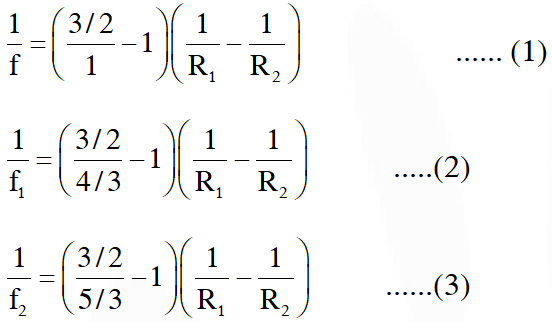Q. A green light is incident from the water to the air - water interface at the critical angle ($\theta$). Select the correct statement. (1) The spectrum of visible light whose frequency is more than that of green light will come out to the air medium. (2) The entire spectrum of visible light will come out of the water at various angles to the normal (3) The entire spectrum of visible light will come out of the water at an angle of$90^{\circ}$to the normal. (4) The spectrum of visible light whose frequency is less than that of green light will come out to the air medium. [JEE-Mains- 2014] Ans. (4) Frequency of light () > frequency of green light ($v_{G}$) µ is also greater than$\mu_{\mathrm{G}}$and critical angle of light is less than green light therefore light will got total internal reflaction and not come out to the air. For frequency of light$(v)
Q. Monochromatic light is incident on a glass prism of angle A. If the refractive index of the material of the prism is $\mu$, a ray, incident at an angle $\theta$, on the face AB would get transmitted through the face AC of the prism provided :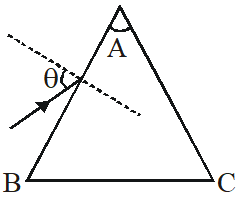$(1) \theta>\cos ^{-1}\left[\mu \sin \left(A+\sin ^{-1}\left(\frac{1}{\mu}\right)\right)\right]$ (2) $\theta<\cos ^{-1}\left[\mu \sin \left(A+\sin ^{-1}\left(\frac{1}{\mu}\right)\right)\right]$ (3) $\theta>\sin ^{-1}\left[\mu \sin \left(A-\sin ^{-1}\left(\frac{1}{\mu}\right)\right)\right]$ (4) $\theta<\sin ^{-1}\left[\mu \sin \left(A-\sin ^{-1}\left(\frac{1}{\mu}\right)\right)\right]$ [JEE-Mains- 2015]
Ans. (3)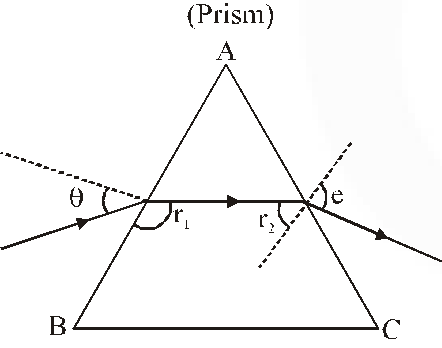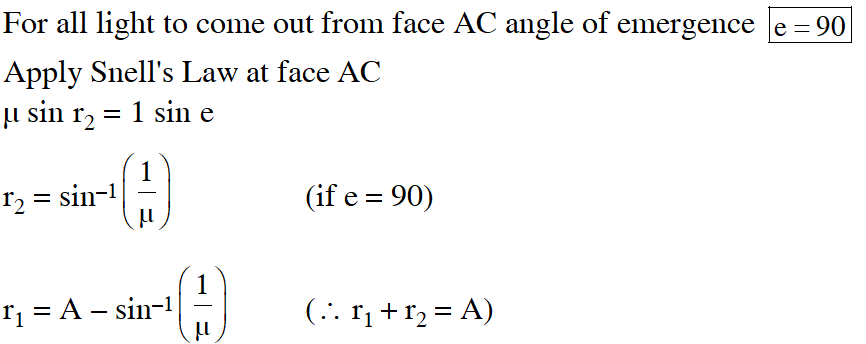Apply Snell's law at face AB $1 \sin \theta=\mu \sin \left(r_{1}\right)$ $\theta=\sin ^{-1}\left(\mu \sin \left(A-\sin ^{-1}\left(\frac{1}{\mu}\right)\right)\right.$ for all light transmitted through $\mathrm{AC}, \mathrm{e}<90^{\circ}$ $\Rightarrow \theta>\sin ^{-1}\left(\mu \sin \left(A-\sin ^{-1}\left(\frac{1}{\mu}\right)\right)\right.$
Q. An observer looks at a distant tree of height 10 m with a telescope of magnifying power of 20. To the observer the tree appears : (1) 20 times nearer (2) 10 times taller (3) 10 times nearer (4) 20 times taller [JEE-Mains- 2016]
Ans. (4) Angular magnification is 20.
Q. In an experiment for determination of refractive index of glass of a prism by i – , plot, it was found that a ray incident at angle $35^{\circ}$, suffers a deviation of $40^{\circ}$ and that it emerges at angle $79^{\circ}$. In that case which of the following is closest to the maximum possible value of the refractive index? (1) 1.8 (2) 1.5 (3) 1.6 (4) 1.7 [JEE-Mains- 2016]
Ans. (2)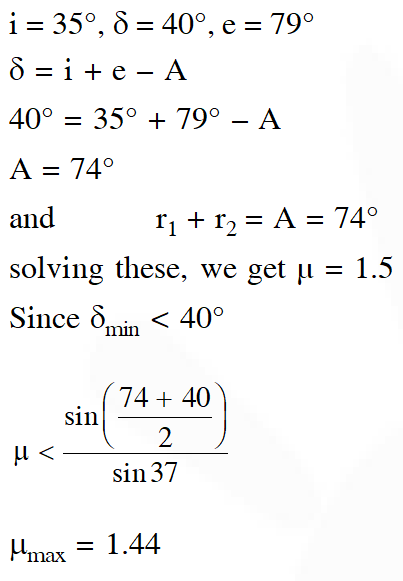Q. A diverging lens with magnitude of focal length 25 cm is placed at a distance of 15 cm from a converging lens of magnitude of focal length 20 cm. A beam of parallel light falls on the diverging lens. The final image formed is : (1) real and at a distance of 40 cm from the divergent lens (2) real and at a distance of 6 cm from the conver (3) real and at a distance of 40 cm from convergent lens (4) virtual and at a distance of 40 cm from convergent lens. [JEE-Mains- 2017]
Ans. (3) As parallel beam incident on diverging lens if forms virtual image at $\mathbf{V}_{1}$ = –25 cm from the diverging lense which works as a object for the converging lense (f = 20 cm)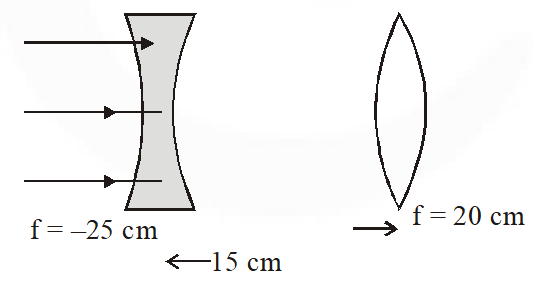So for converging lens u = –40 cm, f = 20 cm $\therefore \quad$ Final image $\frac{1}{\mathrm{V}}-\frac{1}{-40}=\frac{1}{20}$ V = 40 cm from converging lenses.

Chutt
Oct. 13, 2022, 6:04 p.m.
Go
Landd
Oct. 13, 2022, 6:04 p.m.
Confused g
Lund
Oct. 13, 2022, 6:03 p.m.
Costco
Feb. 26, 2022, 11:31 p.m.
Very tricky but nice question to practice
Sombir dixit
April 29, 2021, 4:48 p.m.
Superb Good job, I like it
Jagdish harwani
March 15, 2021, 11:16 a.m.
Copycat of allen
Kr
Feb. 14, 2021, 2:51 p.m.
😁
nahi bataunga
Jan. 2, 2021, 6:13 p.m.
jee is faltu
shushil kumar prajapati
Dec. 8, 2020, 6:31 p.m.
do not open solution
K. Neelima
Dec. 1, 2020, 10:08 a.m.
Tqqq
sumit singh
Oct. 11, 2020, 12:23 p.m.
thankyou very much , it made my work easier and just want to add that keep doing the same .
Jagriti saikia
Sept. 2, 2020, 4:39 p.m.
helpful but need more questions ...and solutions should be more elaborated
Chutar singh
Aug. 29, 2020, 7:54 p.m.
Yeh to shuru hone se pehele hi khatm ho gaya
Aug. 19, 2020, 3:08 p.m.
Mahendra singh dhoni
Aug. 8, 2020, 7:49 p.m.
No proper solution for second question
Avantika
Aug. 4, 2020, 7:37 p.m.
Good , increase the no of questions
Swathi
Aug. 3, 2020, 7:43 p.m.
Increase the number of questions
Harsh
July 16, 2020, 4:49 p.m.
Good and bit tricky questions...liked the questions
Asritha
July 2, 2020, 6:45 p.m.
👏👏👍👍👍 Good..... U gave year wise problems. Solutions can still more better. U increase number of questions. 🙂🙂🙂🙂
D. Tarun
June 25, 2020, 7:57 p.m.
Very helpful but answer 12 should be more clarified because how that equation solved is not understandabl
Atharva
June 14, 2020, 11:05 a.m.
Solutions should be more clear.
ASPIRANT
May 28, 2020, 3:48 p.m.
GOOD even more questions can be provided
Arman
May 4, 2020, 2:48 p.m.
Good .👍👍👍👍......, But only 13 .. Practice needs at least 50-60 ques. And in lockdown books are also not available....
ganesh
May 3, 2020, 12:06 p.m.
good
Vishwa Raghava Reddy
April 10, 2020, 12:38 p.m.
Good